# Hyperbola

A hyperbola is the locus of all those points in a plane such that the difference in their distances from two fixed points in the plane is a constant.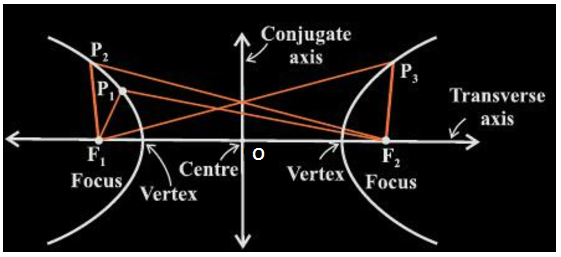The fixed points are referred to as foci (F1 and F2 in the above figure) (singular focus). The above figure represents a hyperbola such that P1F2 – P1F1 = P2F2 – P2F1 = P3F1 – P3F2 is a constant.

When both the foci are joined with the help of a line segment then the mid-point of this line segment joining the foci is known as the center, O represents the center of an ellipse in the figure given above. The line segment passing through both foci is the transverse axis of the hyperbola. The line segment perpendicular to the transverse axis and passing through the center represents the conjugate axis of the hyperbola.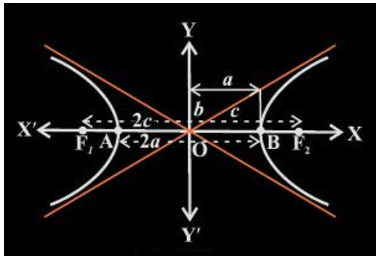The point of intersection of the hyperbola with the transverse axis gives the vertices of the hyperbola represented by the points A and B in the given figure. ‘2a’ denotes the length of the transverse axis. ‘2b’ is the length of the conjugate axis. ‘2c’ represents the distance between the two foci. The relationship between a, b, and c is given by:

b = √(c2 – a2)

## Hyperbola Eccentricity

The ratio of distances from the center of hyperbole from either focus to either of the vertices of the hyperbola is defined as eccentricity.

Eccentricity, e = c/a

Since c ≥ a, the eccentricity is always greater than 1 in the case of a hyperbola.

### Standard Equation of Hyperbola

The simplest method to determine the equation of a hyperbola is to assume that center of the hyperbola is at the origin (0, 0) and the foci lie either on x-axis or y-axis of the Cartesian plane as shown below:

Both the foci lie on x-axis and center O lies at the origin.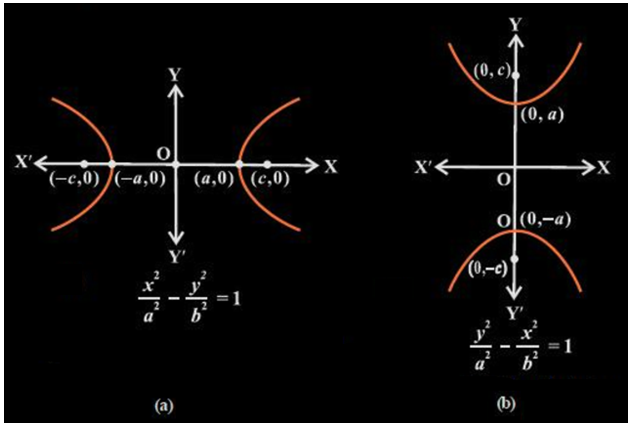Let us consider the figure (a) to derive the equation of hyperbola. Let the co-ordinates of F1 and F2 be (-c, 0) and (c, 0) respectively as shown. Let us consider a point P(x, y) lying on the hyperbola such that P satisfies the definition i.e. the difference of distances of P from F1 and F2 in the plane is a constant 2a.

⇒ PF1 – PF2 = 2a – – – (1)

Using distance formula the distance can be written as: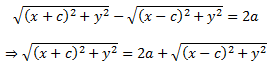Squaring and simplifying both sides we get;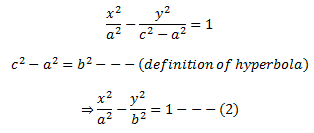Now since P lies on the hyperbola it should satisfy equation (2) such that 0 < a < c.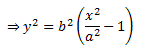Thus,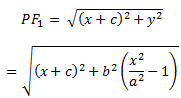On simplifying,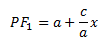Similarly,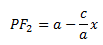In a hyperbola, c > a and because P lies on the right of line x = a, it can be said that x > a  i.e.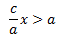Thus PF2 becomes negative.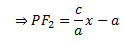Therefore,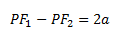Therefore the equation with centre at origin and transverse axis along the x-axis is: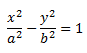Similarly, equation with centre at origin and conjugate axis along the y-axis is:### Latus Rectum of Hyperbola

The line segments perpendicular to the transverse axis through any of the foci such that their endpoints lie on the hyperbola are defined as the latus rectum of a hyperbola.

The length of the latus rectum is 2b2/a.

In this article, we studied the definition, standard equation, eccentricity and latus rectum of a conic section. To understand these concepts and to learn more about conic sections, its types and ellipse with the help of videos, download BYJU’s- The Learning App.